# 详解使用JS如何制作简单的ASCII图与单极图

更新时间：2017年03月31日 08:42:50   投稿：daisy我要评论

ASCII图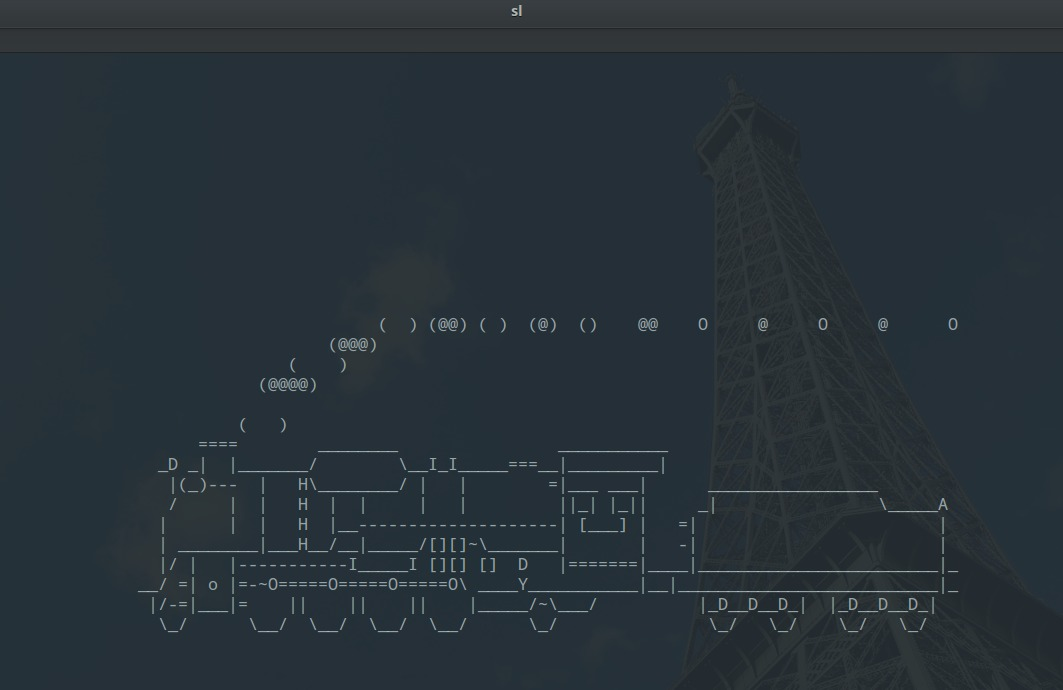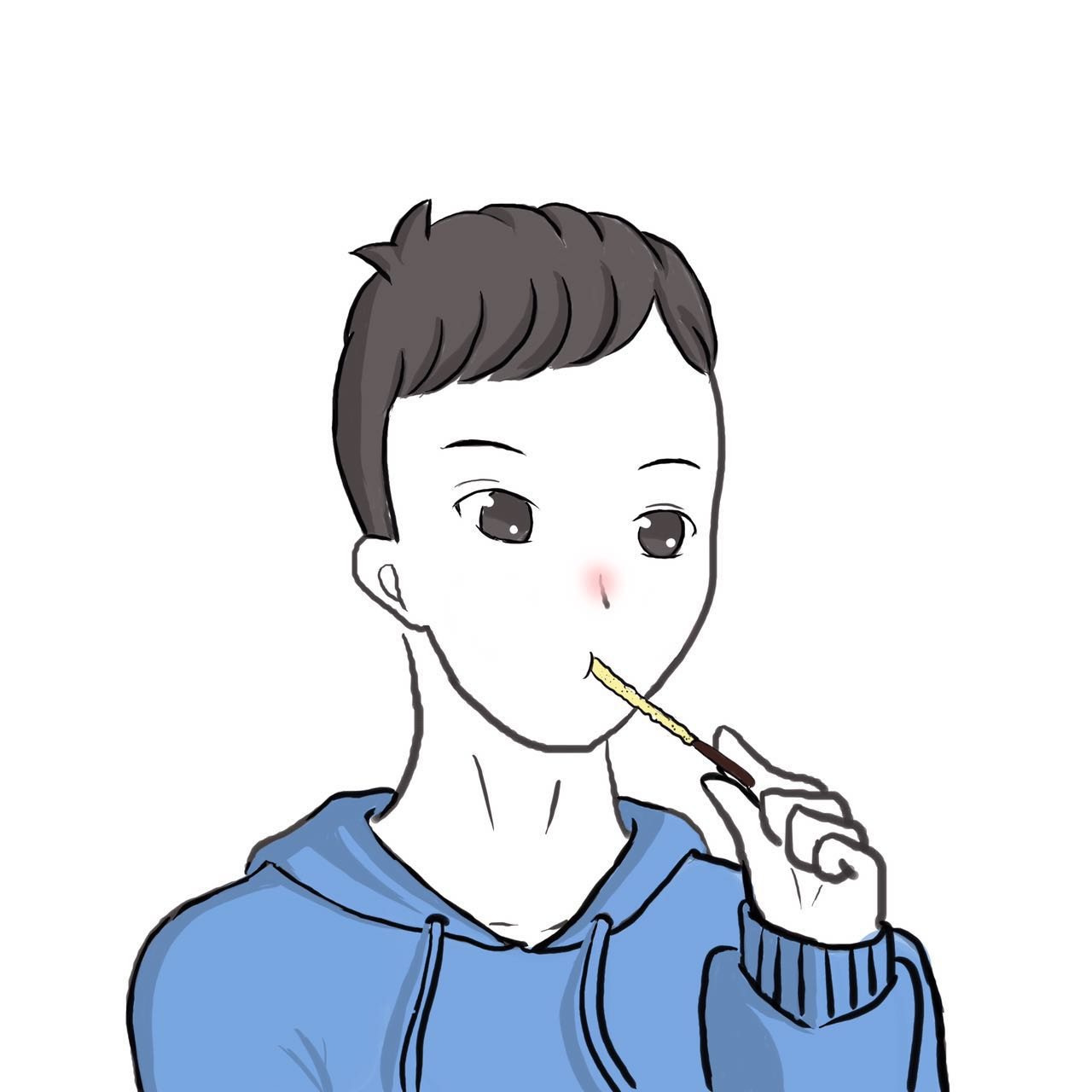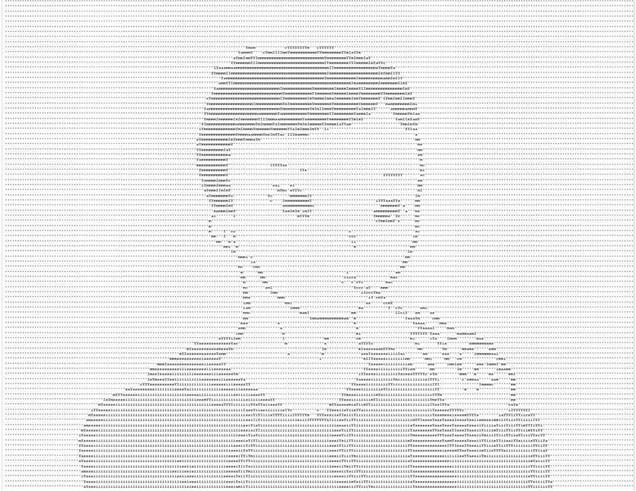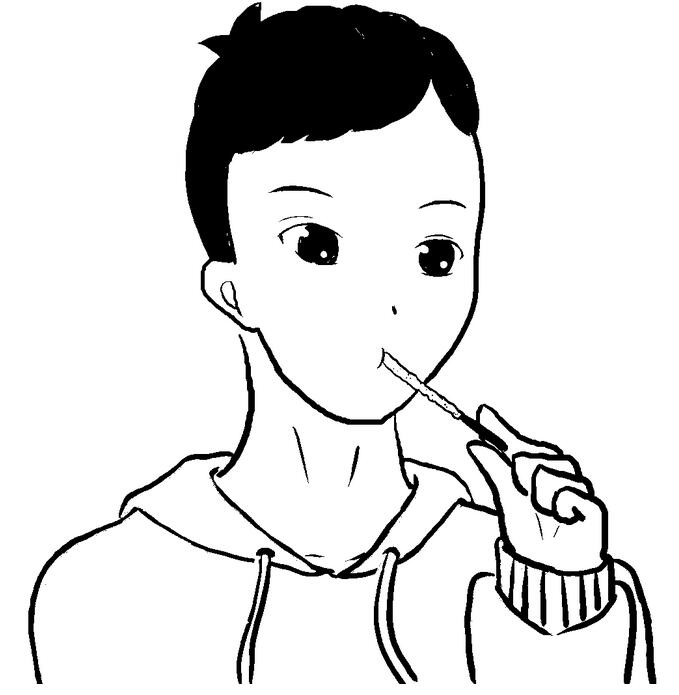• HTML5中的Canvas
• 像素的RGB值
• JS中的Canvas相关API

```var canvasContext = canvas.getContext("2d");
canvasContext.drawImage(sourceImg, 0, 0);
var imgData = canvasContext.getImageData(0 , 0, sourceImg.width, sourceImg.height);
var imgDataArray = imgData.data;```

```var r = imgDataArray[lineIndex];
var g = imgDataArray[lineIndex + 1];
var b = imgDataArray[lineIndex + 2];
var a = imgDataArray[lineIndex + 3];```

Y = (R + G + B) * 1/3

```function rgb2gray(r, g, b) {
return r * 0.333 + g * 0.333 + b * 0.333;
}```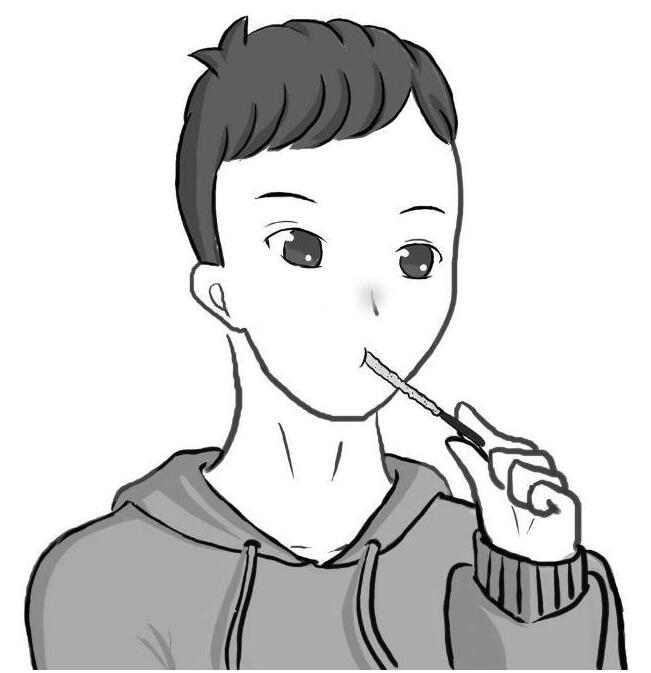```function gray2asc(gray) {
/*ASCII--I'mYasic*/
/*32 64 96 128 160 192 224 256*/
gray = 255 - gray;
if (gray < 128){
if (gray < 64){
if (gray < 32){
return '\''
}
else {
return 'c'
}
}
else {
if (gray < 96){
return 'i'
}
else {
return 's'
}
}
}
else {
if (gray < 192){
if (gray < 160){
return 'I'
}
else {
return 'm'
}
}
else {
if (gray < 224){
return 'a'
}
else {
return 'Y'
}
}
}
}```

``` var result = "";
var lineIndex = 0;
for (var lineHeight = 0; lineHeight < sourceImg.height; lineHeight += 12){
var lineASC = "";
for (var lineFlag = 0; lineFlag < sourceImg.width; lineFlag += 5){
lineIndex = (lineHeight * sourceImg.width + lineFlag) * 4;
var r = imgDataArray[lineIndex];
var g = imgDataArray[lineIndex + 1];
var b = imgDataArray[lineIndex + 2];
lineASC += gray2asc(rgb2gray(r, g, b));
}
lineASC += '\n';
result += lineASC;
}```

``` var canvasContext = targetCanvas.getContext("2d");
canvasContext.drawImage(sourceImg, 0, 0);
var imgData = canvasContext.getImageData(0 , 0, sourceImg.width, sourceImg.height);
var imgDataArray = imgData.data;
for (var index = 0; index <= sourceImg.width * sourceImg.height * 4; index += 4){
var red = imgDataArray[index];
var green = imgDataArray[index + 1];
var blue = imgDataArray[index + 2];
var gray = rgb2gray(red, green, blue);
if (gray < 128){
imgData.data[index] = 0;
imgData.data[index + 1] = 0;
imgData.data[index + 2] = 0;
}
else {
imgData.data[index] = 255;
imgData.data[index + 1] = 255;
imgData.data[index + 2] = 255;
}
}
canvasContext.putImageData(imgData, 0, 0);```

ASCII图完整代码

```function rgb2gray(r, g, b) {
return r * 0.333 + g * 0.333 + b * 0.333;
}

function gray2asc(gray) {
/*ASCII--I'mYasic*/
/*32 64 96 128 160 192 224 256*/
gray = 255 - gray;
if (gray < 128){
if (gray < 64){
if (gray < 32){
return '\''
}
else {
return 'c'
}
}
else {
if (gray < 96){
return 'i'
}
else {
return 's'
}
}
}
else {
if (gray < 192){
if (gray < 160){
return 'I'
}
else {
return 'm'
}
}
else {
if (gray < 224){
return 'a'
}
else {
return 'Y'
}
}
}
}

var img2ASC = function (canvas, sourceImg) {
console.log(sourceImg.width + " " + sourceImg.height);

var canvasContext = canvas.getContext("2d");
canvasContext.drawImage(sourceImg, 0, 0);
var imgData = canvasContext.getImageData(0 , 0, sourceImg.width, sourceImg.height);
var imgDataArray = imgData.data;
var result = "";
var lineIndex = 0;
for (var lineHeight = 0; lineHeight < sourceImg.height; lineHeight += 12){
var lineASC = "";
for (var lineFlag = 0; lineFlag < sourceImg.width; lineFlag += 5){
lineIndex = (lineHeight * sourceImg.width + lineFlag) * 4;
var r = imgDataArray[lineIndex];
var g = imgDataArray[lineIndex + 1];
var b = imgDataArray[lineIndex + 2];
lineASC += gray2asc(rgb2gray(r, g, b));
}
lineASC += '\n';
result += lineASC;
}
document.getElementById("result").innerHTML = result;
};```

```function rgb2gray(r, g, b) {
return r * 0.333 + g * 0.333 + b * 0.333;
}

function gray2asc(gray) {
/*ASCII--I'mYasic*/
/*32 64 96 128 160 192 224 256*/
if (gray < 128){
if (gray < 64){
if (gray < 32){
return '\''
}
else {
return 'c'
}
}
else {
if (gray < 96){
return 'i'
}
else {
return 's'
}
}
}
else {
if (gray < 192){
if (gray < 160){
return 'I'
}
else {
return 'm'
}
}
else {
if (gray < 224){
return 'a'
}
else {
return 'Y'
}
}
}
}

var monoImg = function (targetCanvas, sourceImg) {
targetCanvas.width = sourceImg.width;
targetCanvas.height = sourceImg.height;
var canvasContext = targetCanvas.getContext("2d");
canvasContext.drawImage(sourceImg, 0, 0);
var imgData = canvasContext.getImageData(0 , 0, sourceImg.width, sourceImg.height);
var imgDataArray = imgData.data;
for (var index = 0; index <= sourceImg.width * sourceImg.height * 4; index += 4){
var red = imgDataArray[index];
var green = imgDataArray[index + 1];
var blue = imgDataArray[index + 2];
var gray = rgb2gray(red, green, blue);
if (gray < 128){
imgData.data[index] = 0;
imgData.data[index + 1] = 0;
imgData.data[index + 2] = 0;
}
else {
imgData.data[index] = 255;
imgData.data[index + 1] = 255;
imgData.data[index + 2] = 255;
}
}
canvasContext.putImageData(imgData, 0, 0);
};```